Chapter 5: Exercises

1. (*)Function for KNN search: Write a function knnSearch.m with the following usage:
[index, distance]=knnSearch(x, X, k)
where
• x: an input column vector of $d$-dimension
• X: a dataset matrix of $d$ by $n$, with each column being an observation (data point)
• k: no. of nearest neighbors to be retrieved
• index: a vector of k integers, representing the indices of the first k nearest neighbors (starting from the nearest one to the farthest one)
• distance: a vector of k element, representing the distances to these k nearest neighbors
Hint:
• You can simply assume there is no ties in sorting the distance.
• Distance computation can be achieved by MATLAB command "norm".

Here is a test example:

1. Example 1: knnSearch01.m% Create the datasetn n=30; x=round(rand(n,1)*100); y=round(rand(n,1)*100); X=[x(:), y(:)]'; % Create the data point x=[40, 50]'; % Find the k nearest neighbors k=5; [index, distance]=knnSearch(x, X, k); % Plot the result plot(X(1,:), X(2, :), 'marker', '.', 'linestyle', 'none'); line(x(1), x(2), 'marker', '*'); line(X(1,index), X(2, index), 'marker', 'o', 'color', 'r', 'linestyle', 'none'); box on; axis image fprintf('x=%s\n', mat2str(x)); fprintf('X=%s\n', mat2str(X)); fprintf('k=%s\n', mat2str(k));x=[40;50] X=[73 67 75 20 54 32 39 70 84 44 1 94 85 60 65 17 74 77 15 16 71 40 54 22 4 96 47 62 16 40;69 96 63 62 99 2 4 15 43 80 85 63 10 7 67 54 82 24 81 75 70 35 65 73 71 56 66 38 99 77] k=5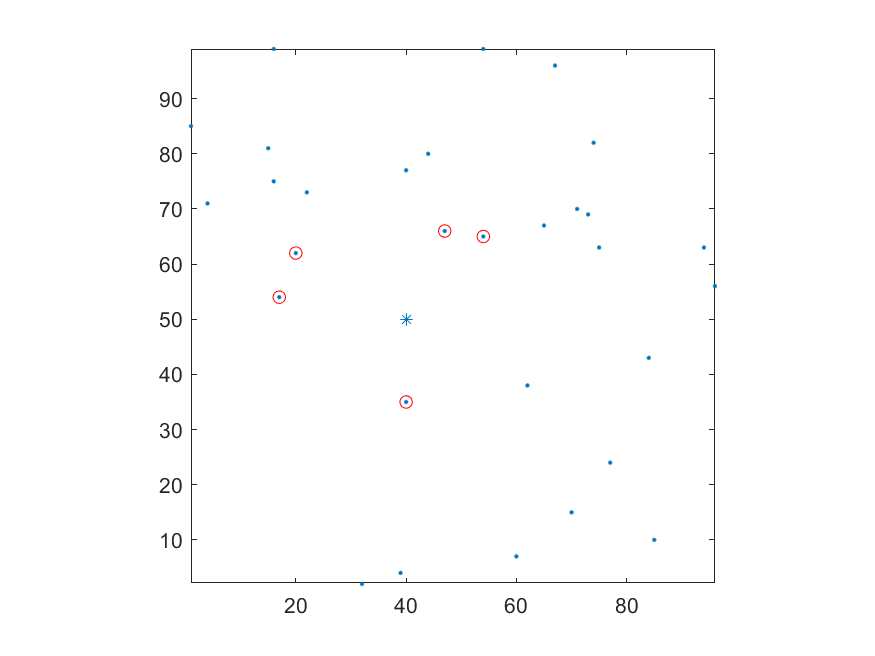2. (*)Function for KNN search via Lp norm: Write a function knnSearchLp.m with the following usage:
[index, distance]=knnSearchLp(x, X, k, p)
where
• x: an input column vector of $d$-dimension
• X: a dataset matrix of $d$ by $n$, with each column being an observation (data point)
• k: no. of nearest neighbors to be retrieved
• p: a positive constant to determine how to compute the distance of Lp norm. Note that $L_p(\mathbf{x})=\left(\sum_{i=1}^d |x_i|^p\right)^{1/p}$.
• index: a vector of k integers, representing the indices of the first k nearest neighbors (starting from the nearest one to the farthest one)
• distance: a vector of k element, representing the distances to these k nearest neighbors
Hint:
• You can simply assume there is no ties in sorting the distance.
• Distance computation can be achieved by MATLAB command "norm".

Here is a test example:

1. Example 2: knnSearchViaLp01.m% Create the datasetn n=30; x=round(rand(n,1)*100); y=round(rand(n,1)*100); X=[x(:), y(:)]'; % Create the data point x=[40, 50]'; fprintf('x=%s\n', mat2str(x)); fprintf('X=%s\n', mat2str(X)); k=5; % Find the k nearest neighbors p=1; [index, distance]=knnSearchViaLp(x, X, k, p); subplot(121); plot(X(1,:), X(2, :), 'marker', '.', 'linestyle', 'none'); title(sprintf('p=%g', p)); line(x(1), x(2), 'marker', '*'); line(X(1,index), X(2, index), 'marker', 'o', 'color', 'r', 'linestyle', 'none'); box on; axis image fprintf('k=%s\n', mat2str(k)); fprintf('p=%s\n', mat2str(p)); p=2; [index, distance]=knnSearchViaLp(x, X, k, p); subplot(122); plot(X(1,:), X(2, :), 'marker', '.', 'linestyle', 'none'); title(sprintf('p=%g', p)); line(x(1), x(2), 'marker', '*'); line(X(1,index), X(2, index), 'marker', 'o', 'color', 'r', 'linestyle', 'none'); box on; axis image fprintf('k=%s\n', mat2str(k)); fprintf('p=%s\n', mat2str(p));x=[40;50] X=[96 9 39 57 30 59 95 40 15 93 27 5 60 31 52 64 45 25 70 10 21 40 36 52 77 23 83 57 85 23;79 89 81 99 17 59 25 85 2 7 63 69 54 90 52 24 22 51 84 11 4 1 26 58 58 26 18 58 57 43] k=5 p=1 k=5 p=2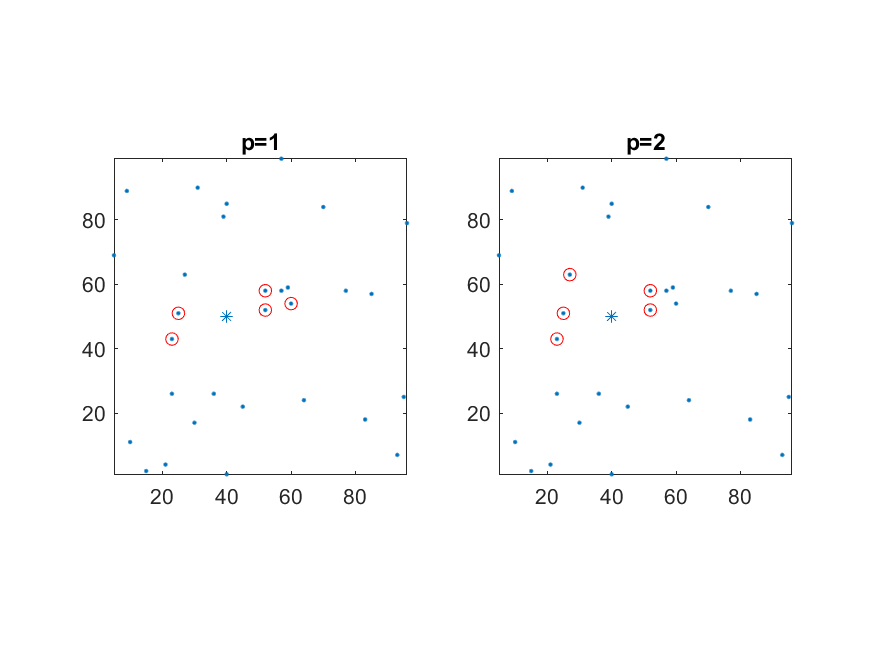1. What is the full name for KNNC?
2. Please describe the basic principle of KNNC.
3. Give the major strength and drawback of KNNC.
4. (*)Voronoi diagram:
1. Given 3 points in a plane, draw its Voronoi diagram.
2. Given 4 points in a plane, draw its Voronoi diagram.
5. (*)Single-layer perceptrons:
1. What is the equation for computing the output of a 2-input single-layer perceptron?
2. What are the learning rules of the 3 parameters in the above equation?
6. (**)Surface and contour plots of 2D Gaussian distributions: Write a script to draw both surface and contour plots of a 2D Gaussian distributions with the following parameters:
1. m = [0, 0]T, S = [1 0; 0 1] (an identity matrix).
2. m = [0, 0]T, S = [1 0; 0 5] (a diagonal matrix).
3. m = [0, 0]T, S = [1 2; 2 5] (a positive-definite matrix).
4. m = [0, 0]T, S = [-1 2; 2 -5]*50 (an arbitrary matrix).
Your plots should be similar to the one shown next: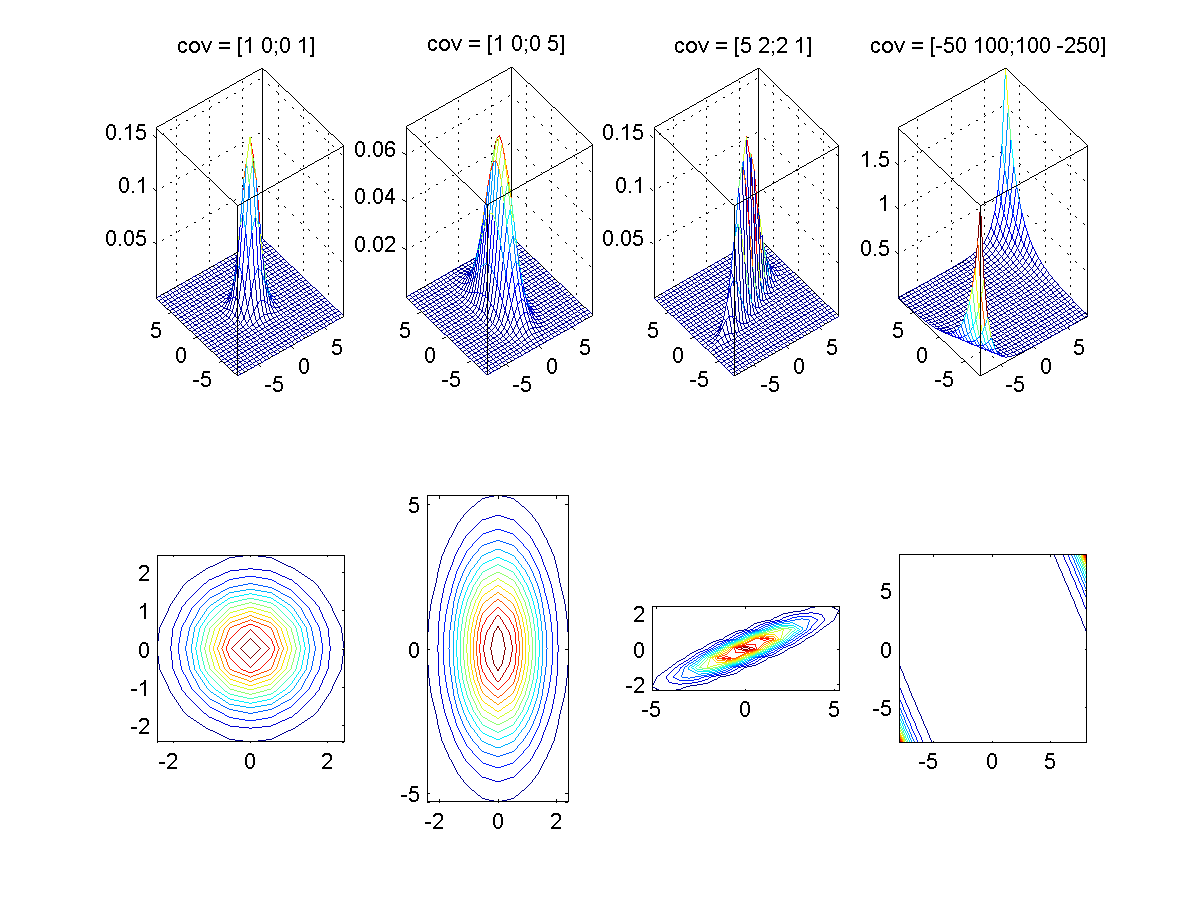You should choose a range that can display important characteristics of these plots. Please explain why the plots of (d) are very different from those of the other cases.
(Hint: You can follow the self demo part of gaussian.m.)
1. Why the classifier is named "quadratic"?
2. How do you train a quadratic classifier?
3. How do you evaluate (test) a quadratic classifier?
4. What is the major strength of a quadratic classifier?
5. What is the major weakness of a quadratic classifier?
6. If a quadratic classifier has a diagonal covariance matrix, does it fall back to a naive Bayes classifier? Why?
8. (*)Naive Bayes classifier:
1. How do you train a naive Bayes classifier?
2. How do you evaluate (test) a naive Bayes classifier?
3. What is the major strength of a naive Bayes classifier?
4. What is the major weakness of a naive Bayes classifier?
9. (**)KNNC on IRIS: recognition rates w.r.t. number of clusters: Please modify the example in order to test the recognition rates of KNNC with respect to the numbers of clusters.
1. Write a script knncIrisRrVsClusterNum01.m to display the recognition rates for both inside and outside tests. Your plot should be similar to the one shown next: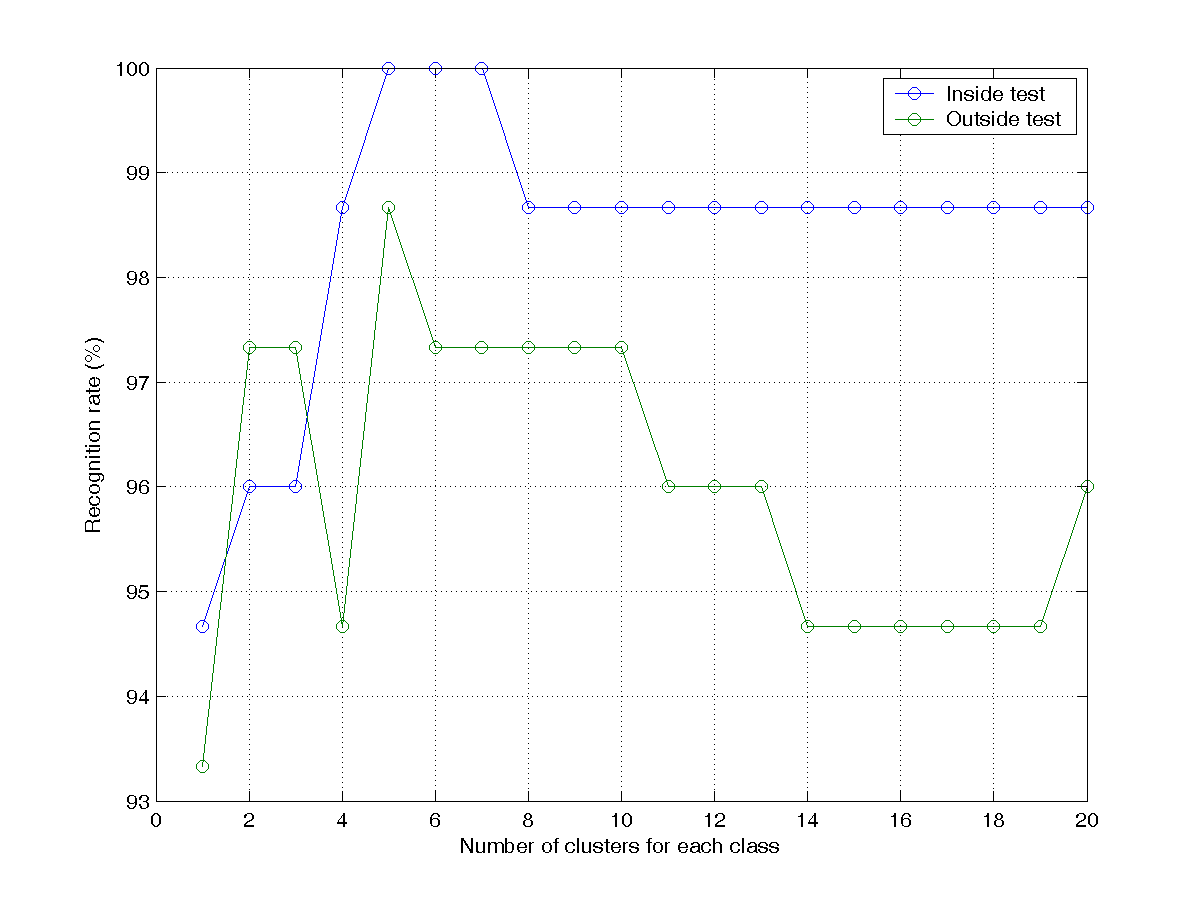This is an example of exhaustive search that can be used to find the best number of clusters for KNNC.
2. Write a script knncIrisRrVsClusterNum02.m that repeats the previous subproblem but reverse the roles of training and test sets. Your plots should be similar to the one shown next: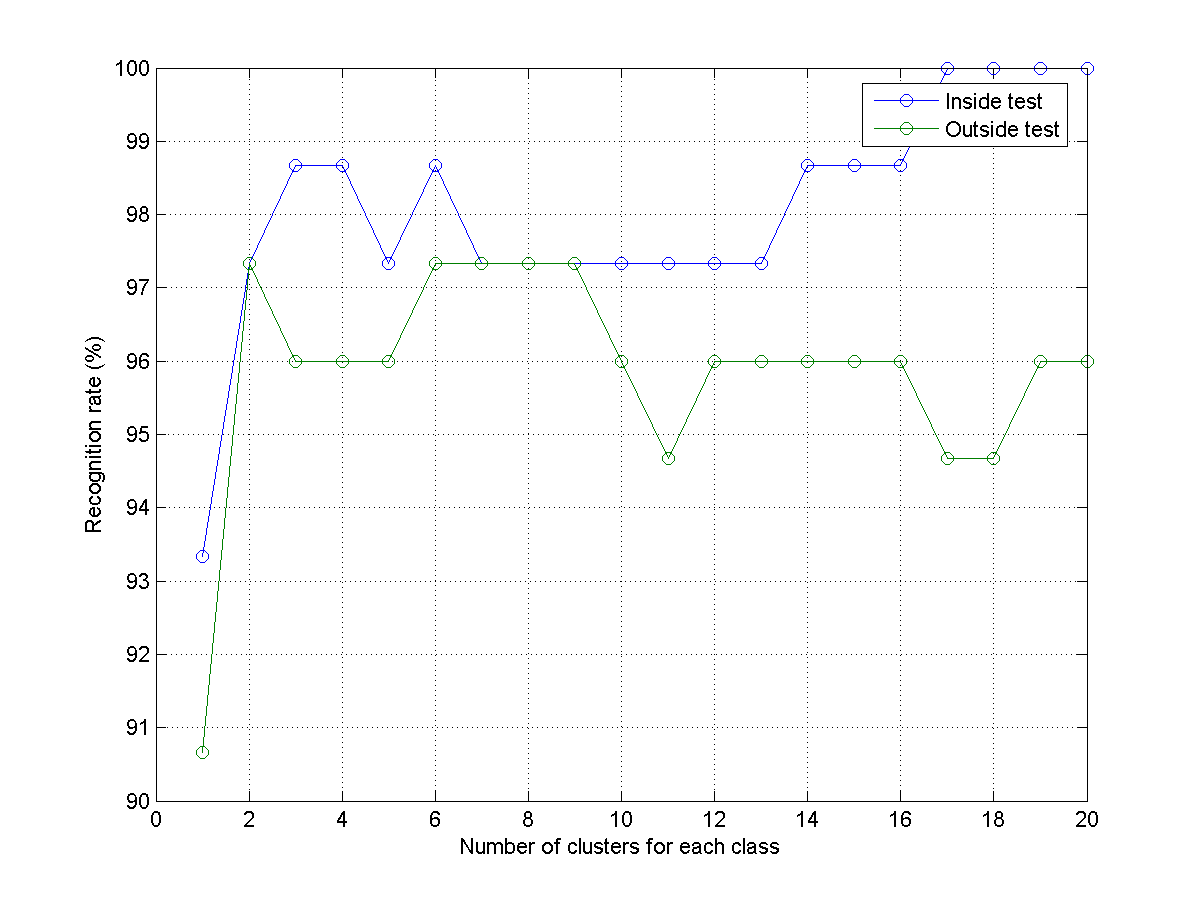3. Write a script knncIrisRrVsClusterNum03.m that combine previous two subproblems to plot the average recognition rates for both inside and outside tests. Your plot should be similar to the one shown next: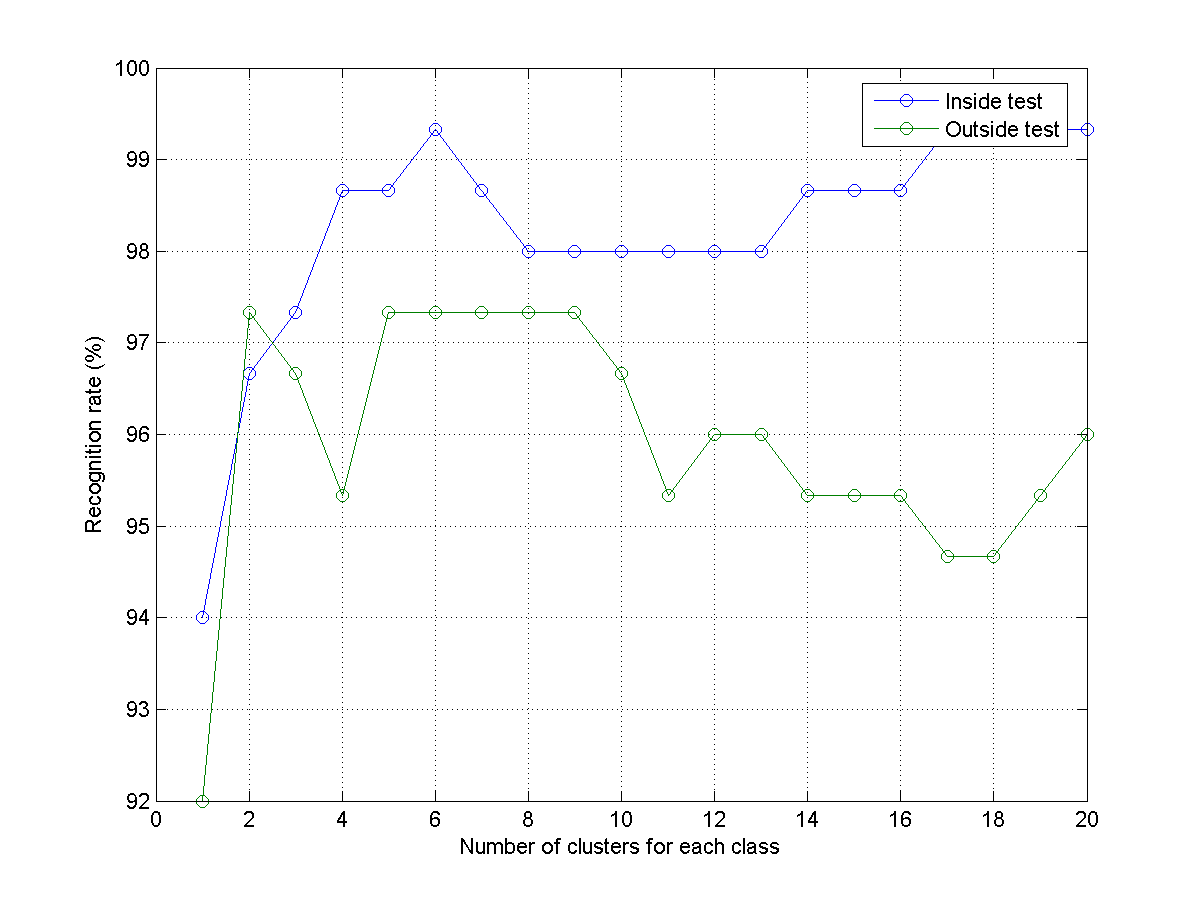This method of switching the role of training and test sets for identify the average recognition rates are referred to as two-fold cross validation. We can extend the concept to K-fold cross validation in which at K-th iteration, only 1/K of the data is for test while the others are for training. This is a more robust method for estimating the performance of the constructed classifier. In general, the inside-test recognition rate should increase with the number of clusters. On the other hand, the outside-test recognition rate should increase initially and then decrease eventually. Usually we take the number of clusters that can maximize the out-side recognition rate for the construction of KNNC classifier.
10. (**)KNNC on WINE: recognition rates w.r.t. number of clusters: Use prData.m to obtain the WINE dataset and repeat the previous exercise to get 3 plots.
11. (*)Various classifiers on WINE dataset: Use prData.m to obtain the WINE dataset. Use the following classifiers to obtain the recognition rates of both inside and outside tests.